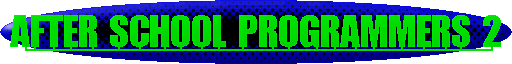Menu Basics Template Images Homework Hexadecimal Text Text and Images Animation Up one level

The last thing we want is to patronise you or offend your knowledge of mathematics, but to understand how Hexadecimal works, you must realise the basics of Decimal to use as a comparison.

Take, for example, 209710. The "10" means "base 10" or decimal. This number can be represented by
2*1000 + 0*100 + 9*10 + 7*1
or more fundamentally,
2*103 + 0*102 + 9*101 + 7*100.

In the same way, a hexadecimal or base 16 number, such as 83116 can be represented by
8*162 + 3*161 + 1*160.
Knowing this, it is easy to convert a hexadecimal number into a decimal number -
(8*162= 8*256= 2048)
(3*161= 3*16=    48)+
(1*160= 1*1=      1)+
=2097
.
There is a long-winded method for converting a decimal number into a hexadecimal number, but you don't need to know it when you have the base converter conveniently found at the bottom of this page!

### Base Converter

To use this converter, enter a numerical value, enter an input base, an output base, and click on "Go!" to obtain a result.
Input value Input base Output base
Output value

You may notice that some of the hexadecimal outcomes contain letters. These letters are only a-f. They represent values larger than 9.
Decimal has ten values - that is, 0, 1, 2, 3, 4, 5, 6, 7, 8 and 9.
In order to represent the extra six values, hexadecimal uses the first six letters of the alphabet, so we have

 Dec Hex 0 1 2 3 4 5 6 7 8 9 10 11 12 13 14 15 0 1 2 3 4 5 6 7 8 9 a b c d e f
If you must convert a hexadecimal value to decimal manually, substitute the decimal values represented by the letters in place of the letters themselves.
"But why is hexadecimal so important?" I hear you ask. When creating a web-page, you need to know hexadecimal for getting the colours of background and text right for your design.

At this point, you should note something which contradicts what you have been taught in art lessons - the primary colours of light are RED, GREEN and BLUE.
"GREEN?" you shout, "Don't you mean YELLOW?"
On a technical note, the three primary colours of pigments are MAGENTA, YELLOW and CYAN, and these are used in any modern colour printer.

To make your lives as programmers easier, here is a colour mixer:

### Paint Mixer

Now that you know how to use this handy program, you need to know how to use the hexadecimal code it produces.
The BODY tag has an attribute called "bgcolor". Notice the American spelling of "color" - there is no "u". To use it, make the tag look like this:
<body bgcolor="#7f7f7f">